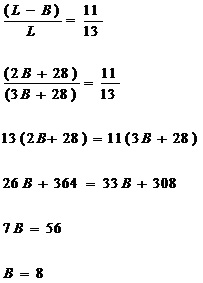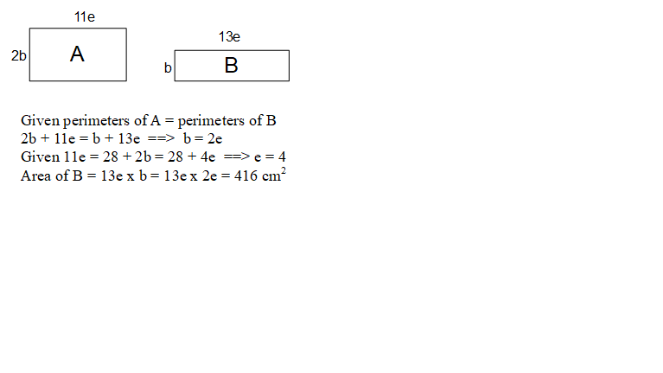# Question

For rectangle ABCD,
length ——- 11u

For rectangle ABCD,
length ——- 13u

11u – 2p ——- 28
(11u + 2p ) x 2 = 22u + 4p ——- (13u + 1p) x 2 = 26u + 2p
26u – 22u = 4u ——- 4p – 2p = 2p
2u ——- 1p
11u – (2 x 2u) = 7u ——- 28
1u ——- 28/7 = 4
13u x 1p ——- 13 x 4 x 2 x 4 = 416

Ans : 416 sq cm.

0 Replies 1 Like ✔Accepted Answer
 Length Breath Perimeter Rectangle ABCD (L – B) 2B (twice of rectangle MNPQ) (L-B) + (L-B) + 2B + 2B = 2L + 2B Rectangle MNPQ L B 2L + 2B (same as rectangle ABCD)

Given: Breath of ABCD is 28cm shorter than its length

(L – B) – 2B = 28

L = 3B + 28

(L – B) = 2B + 28

Given: (L – B) : L = 11 : 13Length of MNPQ, L = 3B + 28 = 24 + 28 = 52 cm

Area of MNPQ = 52 × 8 = 416 cm2

2 Replies 0 Likes0 Replies 2 Likes